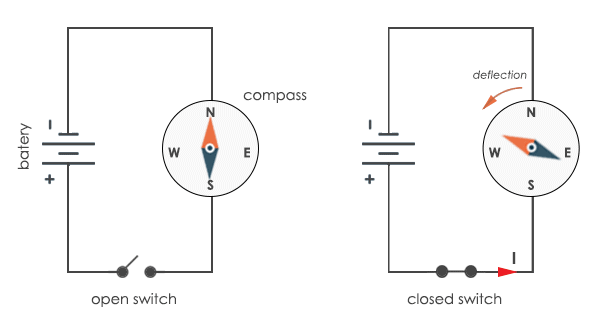# What is Electric Current?

Electricity is a great gift science that has given to mankind. We use electricity almost all the time and it has been a part of our modern lifestyle. We can’t even imagine our lives without this precious gift. Electric current is the core of electricity. And what is electric current? Electric current is the motion of charged particles in wire. For example, a flow of water in river or ocean is called as water current, the same way in electric current, charged particles are flowing.

## Electric charges

There are three particles in an atom; electron, proton, and neutron. Electron is a negatively charged particle while the proton is positively charged. Electrons and protons attract each other while electron-electron (like charges) and proton-proton (like charges) repels each other.

Electric charge particles are electrons and holes. Holes are actually a deficiency of electrons. In a conductor, electrons and holes are present but mostly electrons are there. While in semiconductor both electrons and holes are present in almost equal amount. These charged particles are the basis to make lots of electronic devices like diodes, transistors, etc.

## What is Electric Current?

When an electric conductor is connected across a battery, electric potential is applied to it. This will make the electrons flow from negative terminal to positive terminal of the battery. The free electrons which move while supplying kinetic energy are responsible for conducting property of an element. This movement of electrons which are actually charge carriers produces current. Thus electric current is the rate of flow of electric charge through a conductor with respect to time.

Electric current is denoted with the symbol ‘I‘. Charge of charge carriers is denoted by ‘Q‘.

Therefore, I=Q/t

## Unit of Electric Current

Electric current is the amount of charge passing through a conductor with respect to time. Unit of charge is coulomb and time is seconds. So, the formula for current is given by C/s or we call it Ampere.

1 Ampere of electric current means 1 coulomb of charge passing through a conductor in 1 second.

## Direction of Electric current

When we connect the battery to an electric conductor, electrons from the negative terminal of the battery start moving towards the positive terminal of the battery. Thus the motion of electrons is from negative to positive terminal of the battery. While the direction of conventional current is from positive terminal to negative terminal of the battery.

## Types of Electric Current

Basically, there are two types of current.

Alternating current: Alternating current (AC) is the flow of charges that changes its direction periodically. This type of supply is used to power buildings, offices, etc. You get the AC power supply from the mains socket at your home.

Direct current: Direct current (DC) is the flow of charges in only one direction. In DC source, electrons emerge from negative terminal and move towards positive terminal of the battery. We power most of the low power devices with DC power. If we want to supply these devices from the mains socket, you need to convert it into Direct Current (DC) supply first.

## Effects of Electric current

There are mainly two effects of electric current:

1. Heat effect: Current is dissipated in the form of heat. Heat evolved is directly proportional to the current flowing. If the current flowing is less, heat will be less and if the current flowing is more, more amount of heat is produced.

This heating effect is useful in several ways. This heating effect is used in electric heater. Here resistance is increased to increase the amount of heat. Moreover, it is also used in the electric fuse. The more heat will break the fuse wire and thus circuit will break.

2. Magnetic effect: We can observe magnetic field across a current carrying wire. You can place a compass near an electrically stressed conductor and you will observe deflection in compass. This shows the presence of a magnetic field. This is the well-known Oersted’s experiment.That’s all about electric current. Now you can understand how important electric current is in our day to day life and of course, it is not possible to assume this modern world without electricity. But it is important to know where it all started with the study of electric current. Today current is used to transfer data and it’s just a minute amount of current as compared to light bulb. We use internet connection, Ethernet and other things that are based on the information carrying current. Current is becoming more and more important to our lives day by day.